# 一段因 @State 注入机制所产生的“灵异代码”

## 问题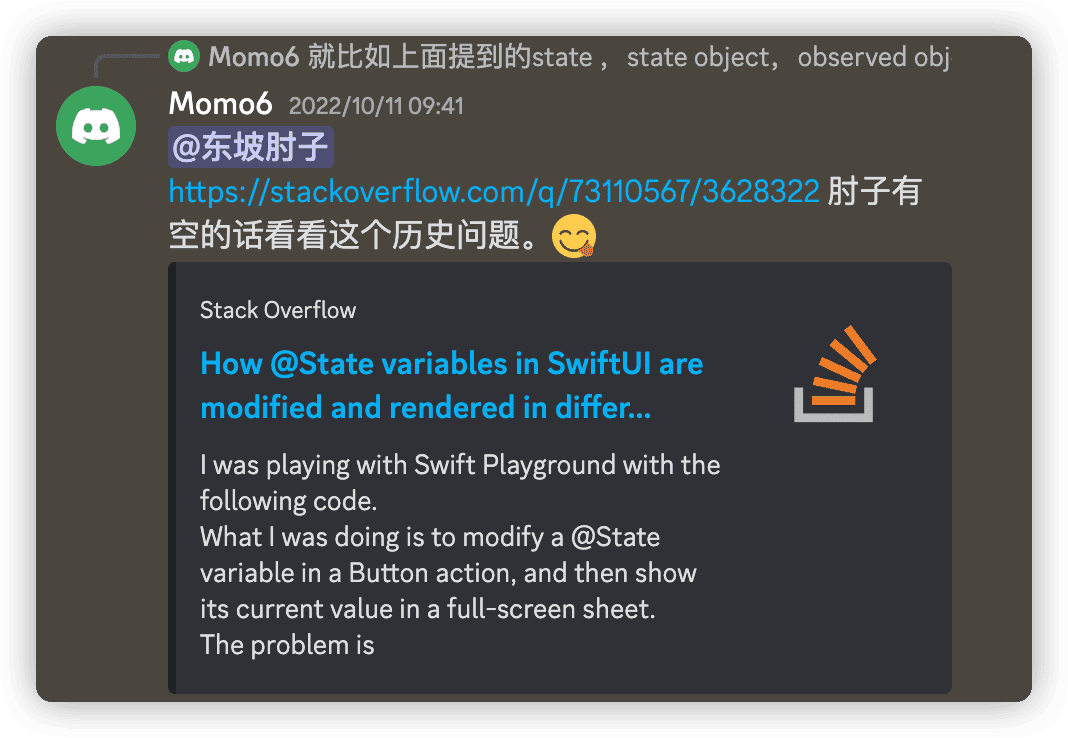``````struct ContentView: View {
@State private var n = 1
@State private var show = false

var body: some View {
VStack {
// 如果注释掉下面这行 Text 代码
// 在按下 Button ( n = 2 ) 后 , full-screen 中的 Text 仍显示 n = 1

// Text("n = \(n)") // 解除注释，sheet 中的 Text 将显示 n = 2

Button("Set n = 2") {
n = 2
show = true
}
}
.fullScreenCover(isPresented: \$show) {
VStack {
Text("n = \(n)")
Button("Close") {
show = false
print("n in fullScreenCover is", n) // 无论是否注释掉上面的 Text ，此处均打印为 2
}
}
}
}
}
``````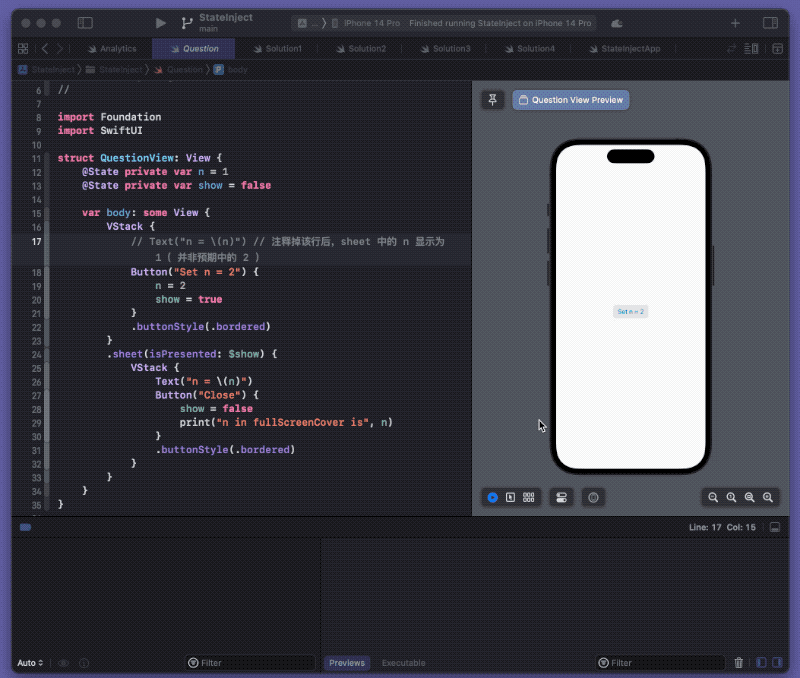## 问题构成

### State 注入的优化机制

``````struct StateTest: View {
@State var n = 10
var body: some View {
VStack {
let _ = print("update")
Text("Hello")
Button("n = n + 1") {
n += 1
print(n)
}
}
}
}
``````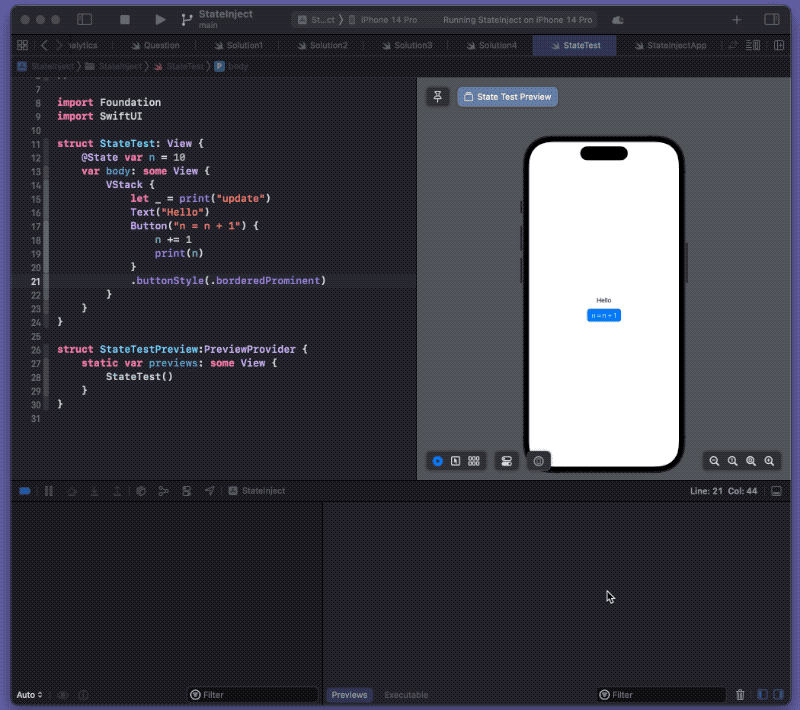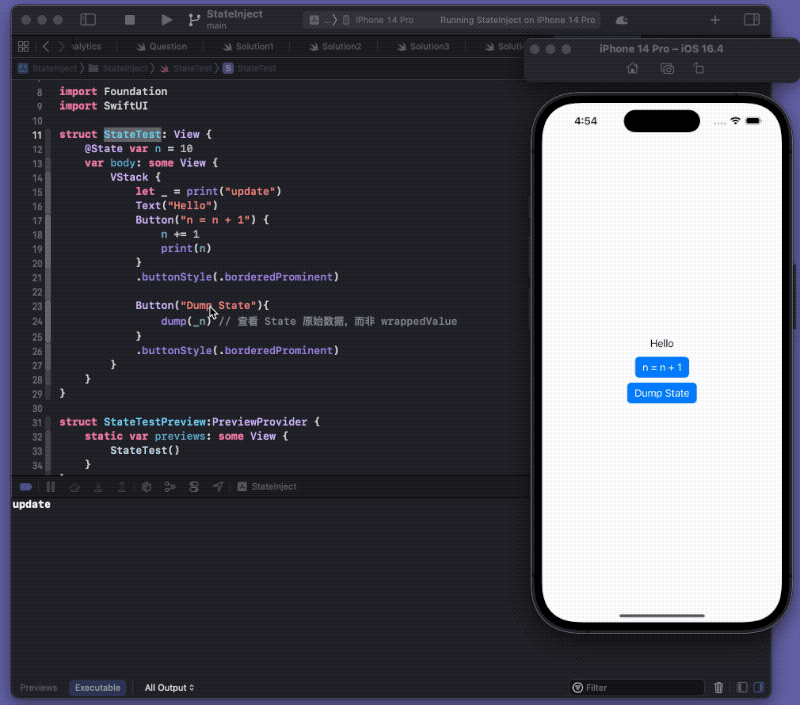``````struct ContentView: View {
@State private var n = 1
@State private var show = false

var body: some View {
VStack {
// Text("n = \(n)") // 注释掉该行后，sheet 中的 n 显示为 1（ 并非预期中的 2 ）
Button("Set n = 2") {
n = 2
show = true
}
.buttonStyle(.bordered)
}
.sheet(isPresented: \$show) {
VStack {
Text("n = \(n)")
Button("Close") {
show = false
print("n in fullScreenCover is", n)
}
.buttonStyle(.bordered)
}
}

}
}
``````

### Sheet（ FullScreenCover ）视图的生命周期

• overlay、background 等，会在 body 求值时调用、解析（ 因为要与主视图一并显示 ）

``````struct AnalyticsView: View {
@State private var n = 1
@State private var show = false

var body: some View {
let _ = print("Parent View update") // 主视图 body 求值
VStack {
// Text("n = \(n)") // 注释掉该行后，sheet 中的 n 显示为 1（ 并非预期中的 2 ）
Button("Set n = 2") {
n = 2
show = true
}
.buttonStyle(.bordered)
}
.sheet(isPresented: \$show) {
SheetInitMonitorView(show: \$show, n: n)
}
}
}

struct AnalyticsViewPreview: PreviewProvider {
static var previews: some View {
AnalyticsView()
}
}

struct SheetInitMonitorView: View {
@Binding var show: Bool
let n: Int
init(show: Binding<Bool>, n: Int) {
self._show = show
self.n = n
print("sheet view init") // 创建实例（ 表示 sheet 的闭包被调用 ）
}

var body: some View {
let _ = print("sheet view update") // sheet 视图求值
VStack {
Text("n = \(n)")
Button("Close") {
show = false
print("n in fullScreenCover is", n)
}
.buttonStyle(.bordered)
}
}
}
``````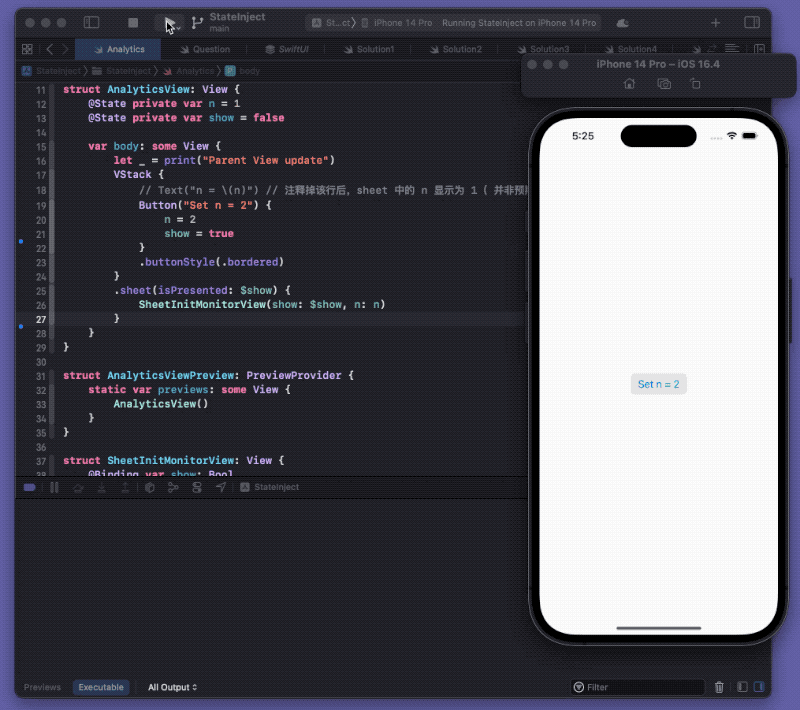``````.fullScreenCover(isPresented: \$show) {
VStack {
Text("n = \(n)")
Button("Close") {
show = false
print("n in fullScreenCover is", n) // 无论是否注释掉上面的 Text ，此处均打印为 2
}
}
}
``````

``````Button("Set n = 2") {
n = 2
show = true
DispatchQueue.main.asyncAfter(deadline: .now() + 0.1){ // 延迟已保证 Sheet 中的视图已完成创建
dump(_n)
}
}
``````

## 现象分析

### 当 ContextView 中不包含 Text（ ContextView 没有与 n 创建关联 ）

• 程序运行，SwiftUI 对 ContextView 的 body 进行求值并渲染
• `.fullScreenCover` 的闭包此时并未被调用，但捕获了视图当前的 n 值 （ n = 1 ）
• 点击 Button 后，尽管 n 的内容发生变化，但 ContextView 的 body 并未重新求值
• 由于 show 转变为 true ，SwiftUI 开始调用 `.fullScreenCover` 的闭包，创建 Sheet 视图

• 由于合并操作的原因，在 Sheet 视图关联到 n 后，并不会重新更新
• Sheet 中的 Text 显示 n = 1
• 点击 Sheet 中的 Close 按钮，执行 Button 闭包，重新获得 n 的当前值（ n = 2 ），打印值为 2

### 当 ContextView 中包含 Text （ ContextView 与 n 之间创建了关联 ）

• 程序运行，SwiftUI 对 ContextView 的 body 进行求值并渲染
• `.fullScreenCover` 的闭包此时并未被调用，但捕获了视图当前的 n 值 （ n = 1 ）
• 点击 Button 后，由于 n 值发生了变化，ContextView 重新求值（ 重新解析 DSL 代码 ）
• 在重新求值的过程中，`.fullScreenCover` 的闭包捕获了新的 n 值 （ n = 2 ）
• 创建 Sheet 视图并渲染
• 由于 `.fullScreenCover` 闭包已经毕竟捕获了新值，因此 Sheet 的 Text 显示为 n = 2

## 解决方案

### 方案一、在 DSL 中进行关联，强制刷新

``````Button("Set n = 2") {
n = 2
show = true
}
.buttonStyle(.bordered)
// .id(n)
.onChange(of:n){_ in } // id 或 onChange 均可以在不添加显示内容的情况下，创建关联
``````

### 方案二、使用 @StateObject 强制刷新

``````struct Solution2: View {
@StateObject var vm = VM()
@State private var show = false

var body: some View {
VStack {
Button("Set n = 2") {
vm.n = 2
show = true
}
.buttonStyle(.bordered)
}
.sheet(isPresented: \$show) {
VStack {
Text("n = \(vm.n)")
Button("Close") {
show = false
print("n in fullScreenCover is", vm.n)
}
.buttonStyle(.bordered)
}
}
}
}

class VM: ObservableObject {
@Published var n = 1
}
``````

### 方案三、使用 Binding 类型，重获新值

Binding 中 get 方法对应的是 ContextView 中 n 的原始地址，无需经过为 Sheet 重新注入的过程，因此在求值阶段便可以获得最新值

``````struct Solution3: View {
@State private var n = 1
@State private var show = false

var body: some View {
VStack {
Button("Set n = 2") {
n = 2
show = true
}
.buttonStyle(.bordered)
}
.sheet(isPresented: \$show) {
SheetView(show: \$show, n: \$n)
}
}
}

struct SheetView:View {
@Binding var show:Bool
@Binding var n:Int
var body: some View {
VStack {
Text("n = \(n)")
Button("Close") {
show = false
print("n in fullScreenCover is", n)
}
.buttonStyle(.bordered)
}
}
}
``````

### 方案四、延迟更新数据

``````struct Solution4: View {
@State private var n = 1
@State private var show = false

var body: some View {
VStack {
Button("Set n = 2") {
// 极小的延迟便可以达到效果
n = 2
}
show = true
}
.buttonStyle(.bordered)
}
.sheet(isPresented: \$show) {
VStack {
Text("n = \(n)")
Button("Close") {
show = false
print("n in fullScreenCover is", n)
}
.buttonStyle(.bordered)
}
}
}
}
``````

## 总结

 用 SwiftUI 的方式进行布局 自定义 Button 的外观和交互行为